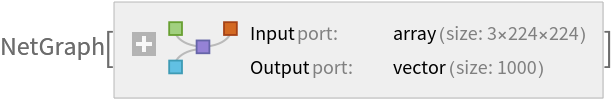# YOLO V8 Classify Trained onImageNet Competition Data

Identify the main object in an image

YOLO (You Only Look Once) Version 8 by Ultralytics is the latest version of the YOLO models. Just like its predecessor, YOLO Version 5, YOLO Version 8 is an anchor-free model that was trained with mosaic augmentation. It features the use of new "C2f" blocks, which employ additional dense connections between bottleneck modules. Although YOLO models are historically object detection models, in this case, their heads were adapted for image classification and the models were trained on ImageNet.

## Model Information

•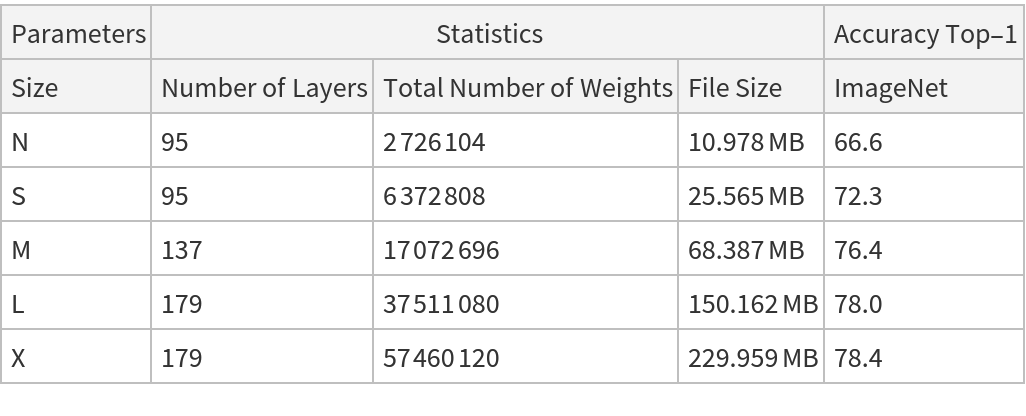## Examples

### Resource retrieval

Get the pre-trained net:

 In:=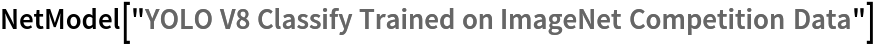Out=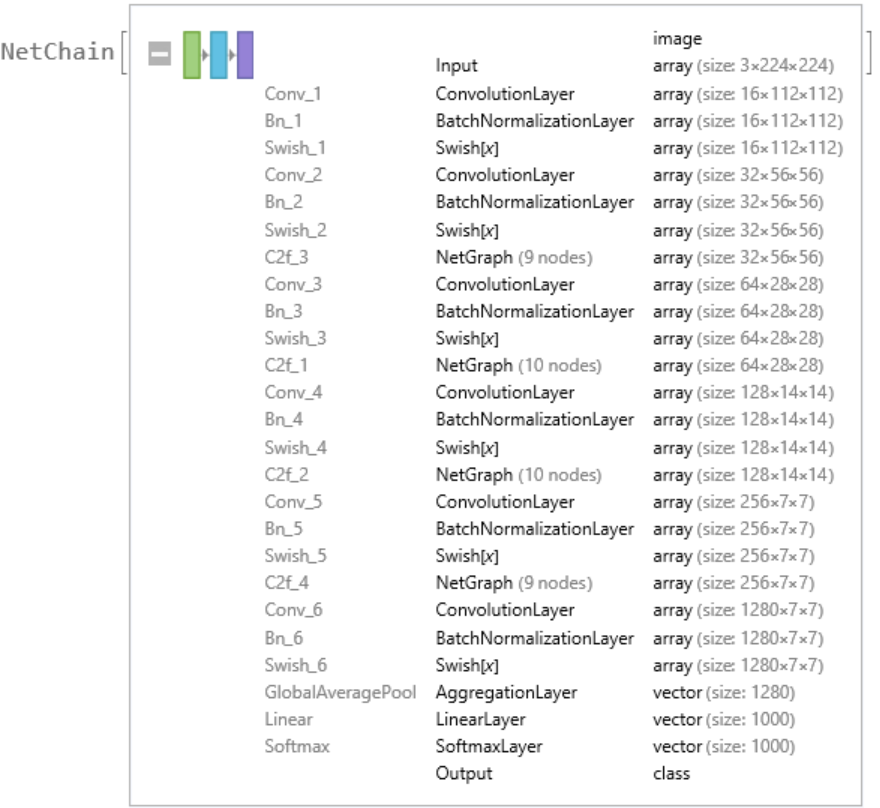### NetModel parameters

This model consists of a family of individual nets, each identified by a specific parameter combination. Inspect the available parameters:

 In:=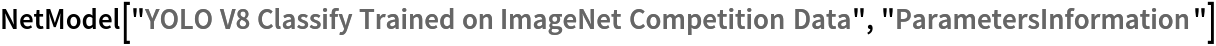Out=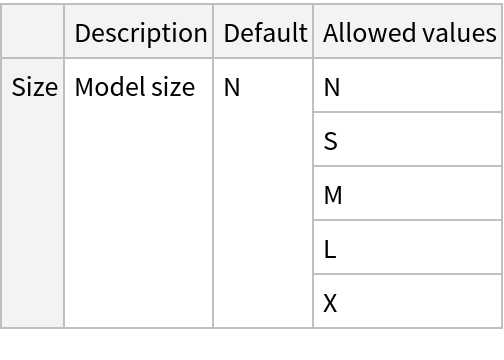Pick a non-default net by specifying the parameters:

 In:=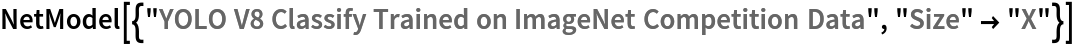Out=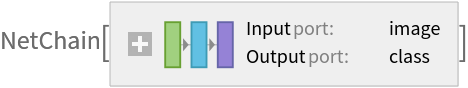Pick a non-default uninitialized net:

 In:=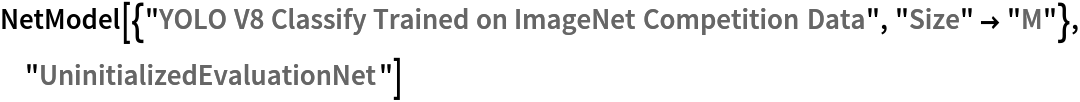Out=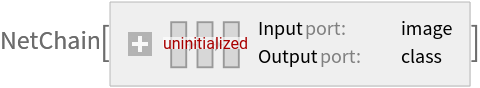### Basic usage

Classify an image:

 In:=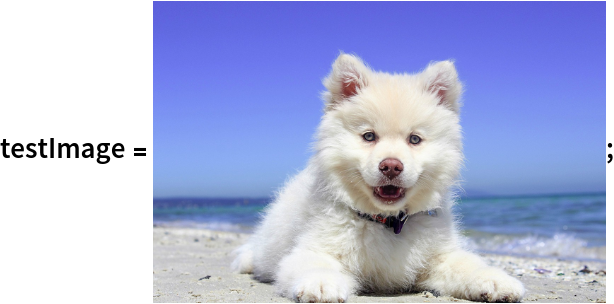In:=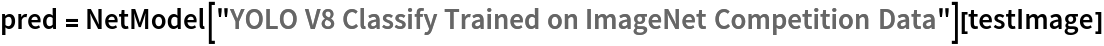Out=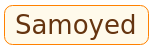The prediction is an Entity object, which can be queried:

 In:=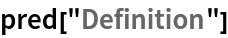Out=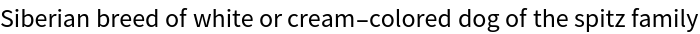Get a list of available properties of the predicted Entity:

 In:=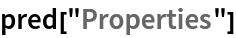Out=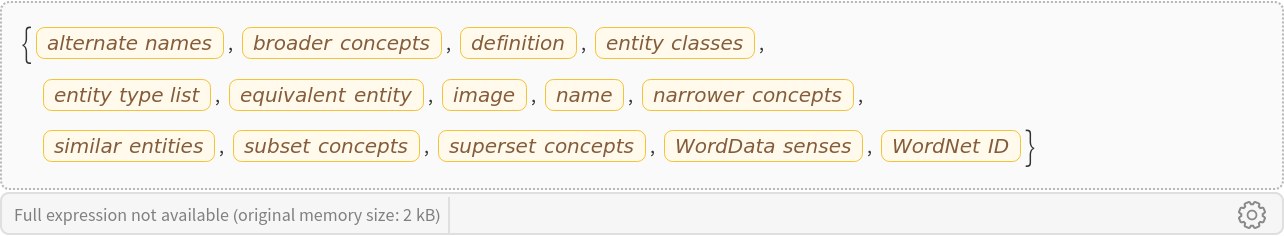Obtain the probabilities of the 10 most likely entities predicted by the net:

 In:=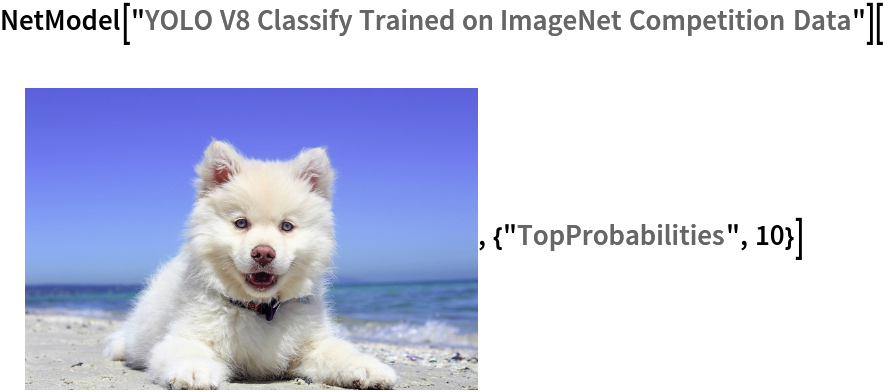Out=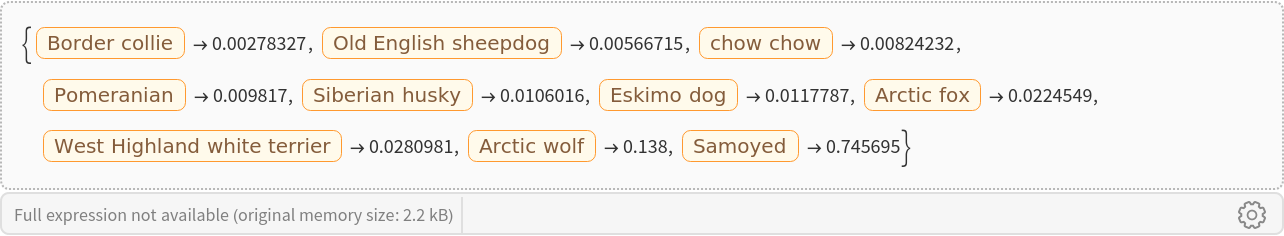An object outside the list of the ImageNet classes will be misidentified:

 In:=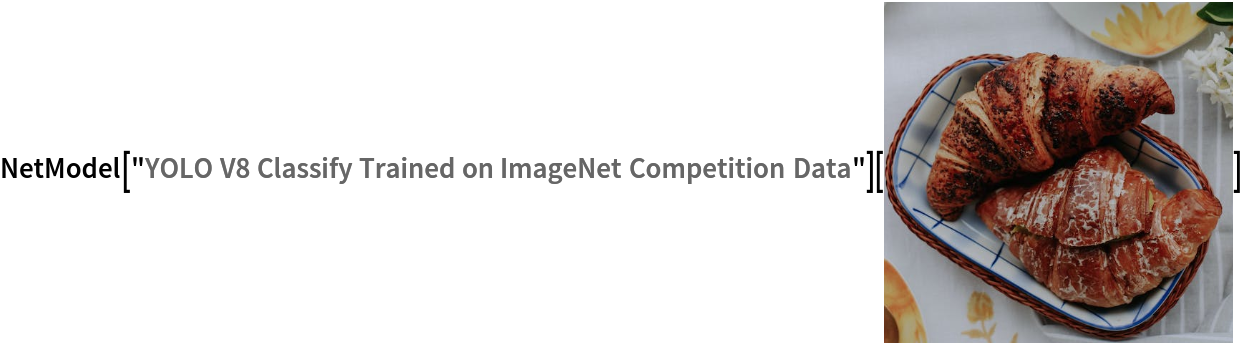Out=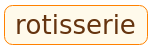Obtain the list of names of all available classes:

 In:=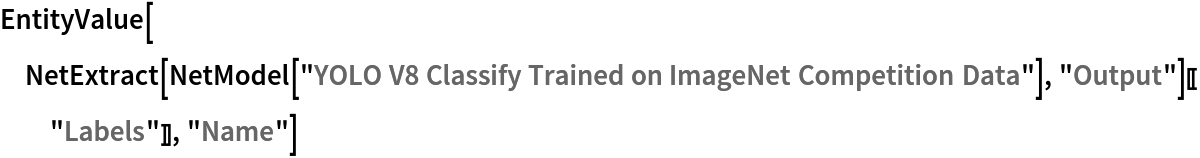Out=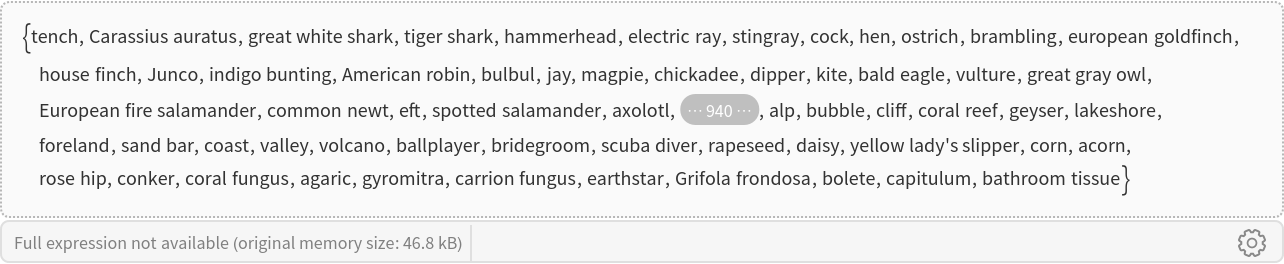### Feature extraction

Remove the last two layers of the trained net so that the net produces a vector representation of an image:

 In:=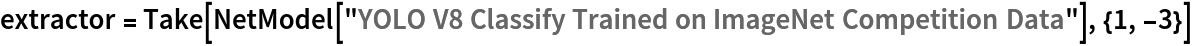Out=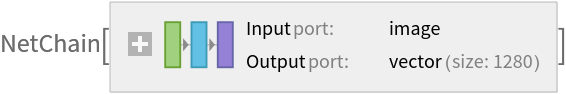Get a set of images:

 In:=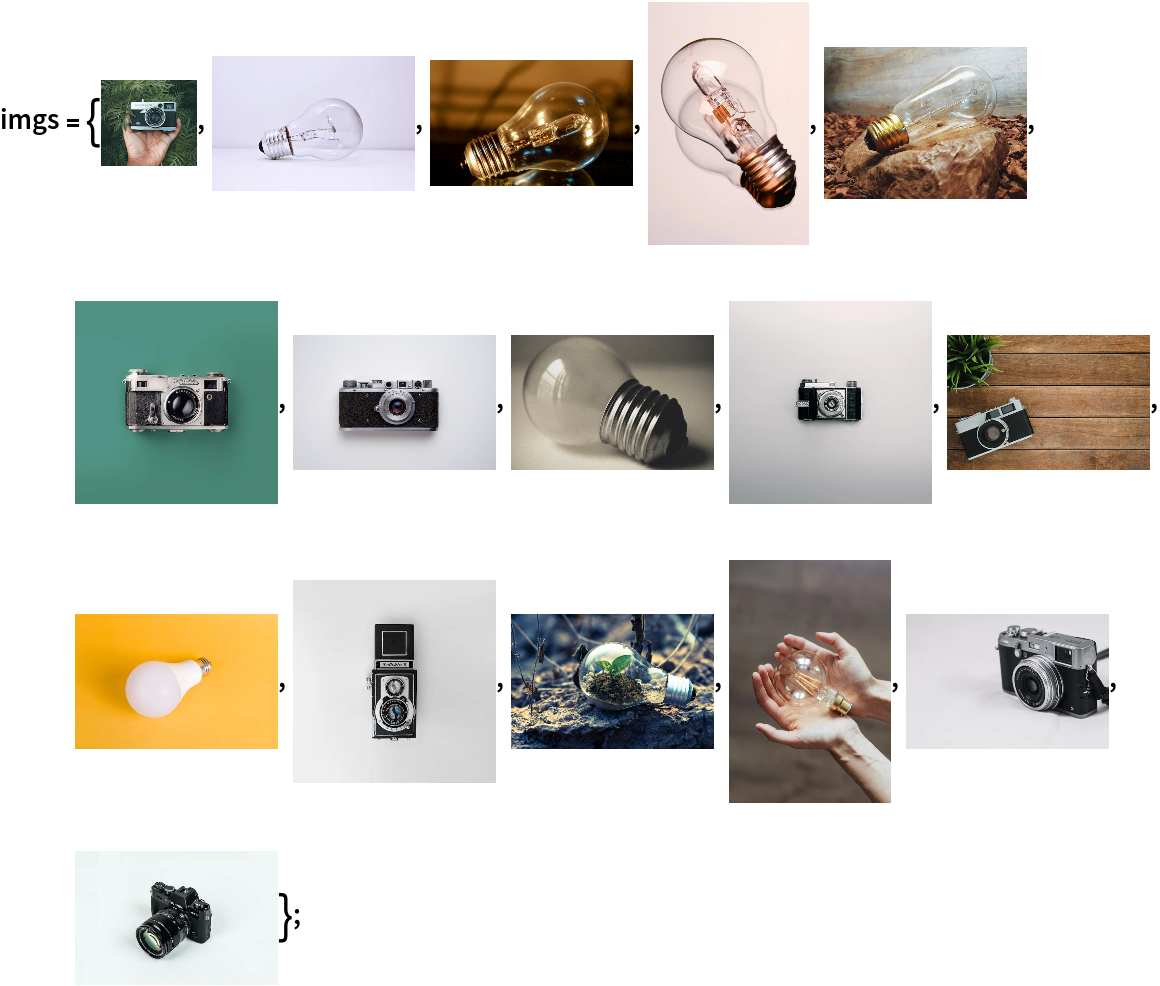In:=Visualize the features of a set of images:

 In:=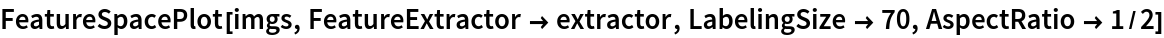Out=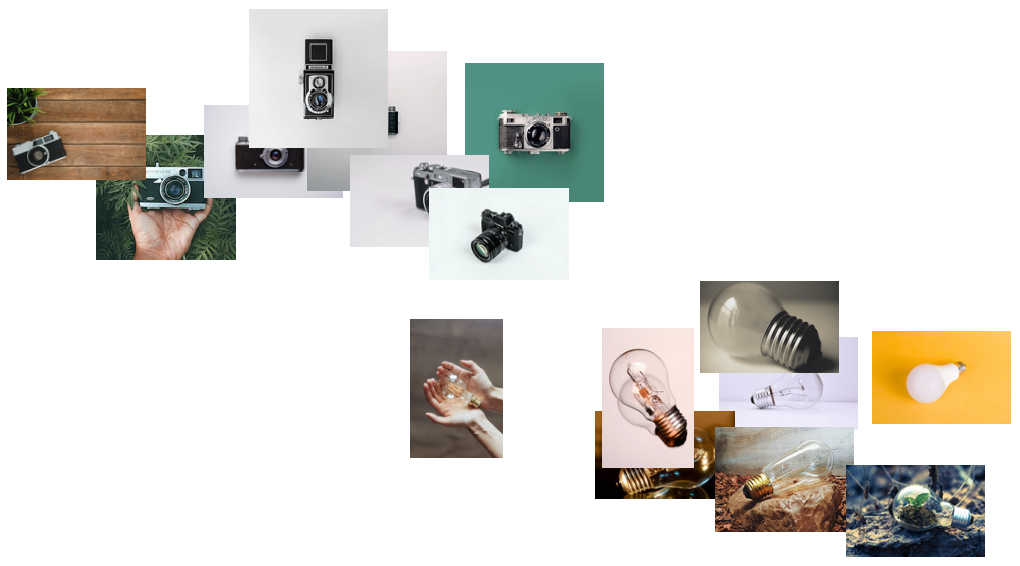### Transfer learning

Use the pre-trained model to build a classifier for telling apart images of bulbs and cameras. Create a test set and a training set:

 In:=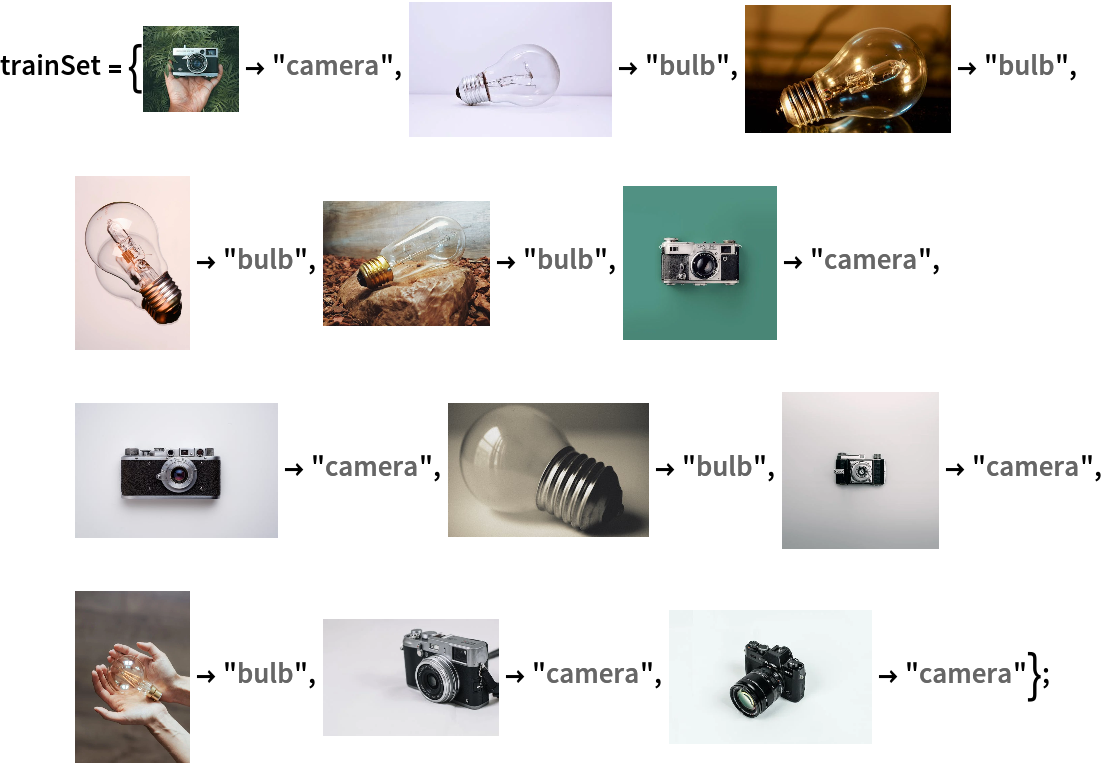In:=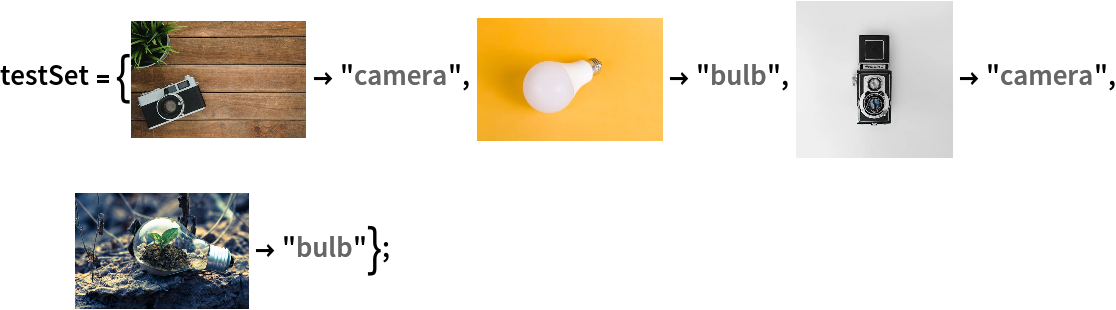Remove the last classification layers from the pre-trained net:

 In:=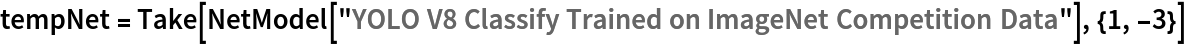Out=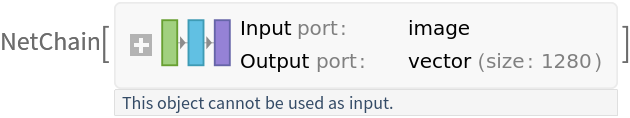Create a new net composed of the pre-trained net followed by a linear layer and a softmax layer:

 In:=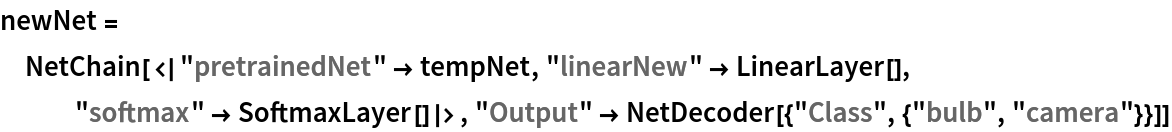Out=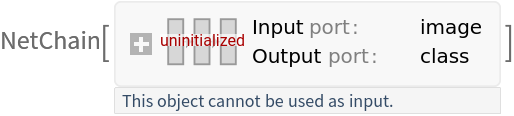Train on the dataset, freezing all the weights except for those in the "linearNew" layer (use TargetDevice -> "GPU" for training on a GPU):

 In:=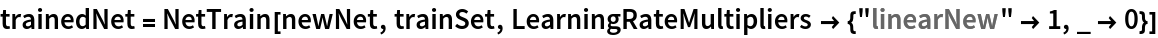Out=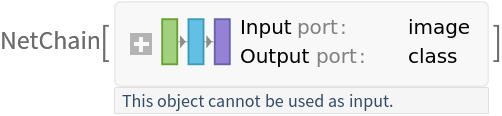Perfect accuracy is obtained on the test set:

 In:=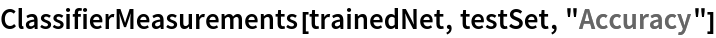Out=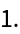### Net information

Inspect the number of parameters of all arrays in the net:

 In:=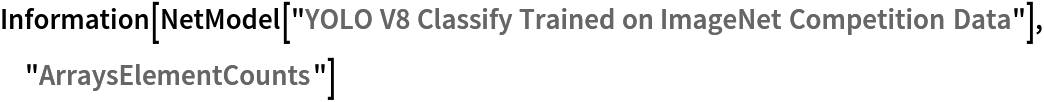Out=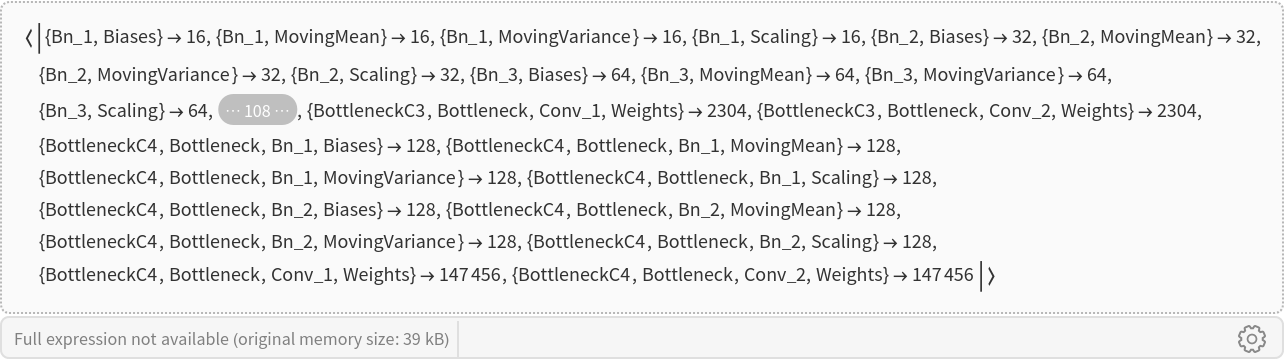Obtain the total number of parameters:

 In:=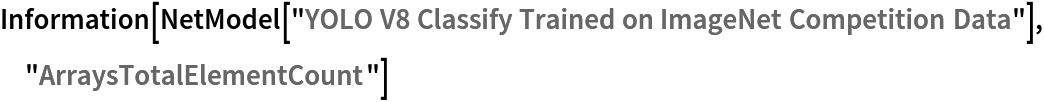Out=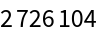Obtain the layer type counts:

 In:=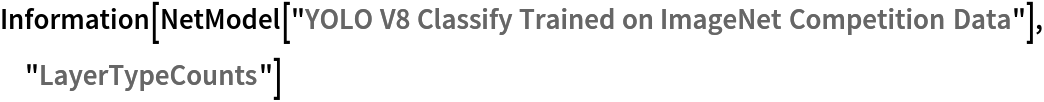Out=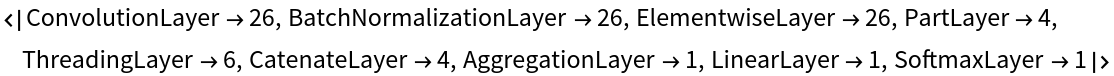Display the summary graphic:

 In:=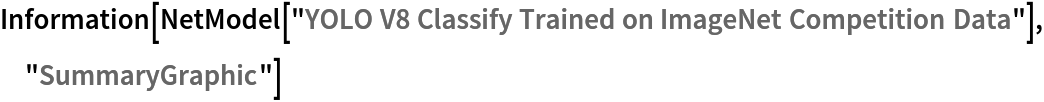Out=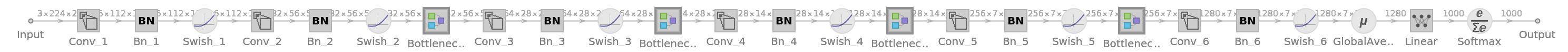### Export to ONNX

Export the net to the ONNX format:

 In:=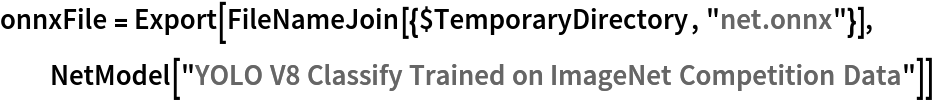Out=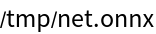Get the size of the ONNX file:

 In:=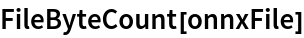Out=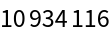The size is similar to the byte count of the resource object:

 In:=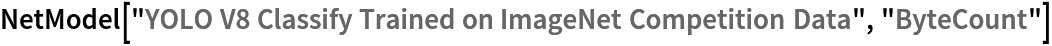Out=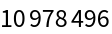Check some metadata of the ONNX model:

 In:=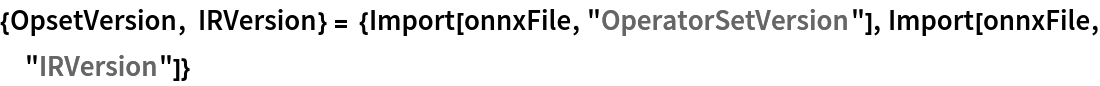Out=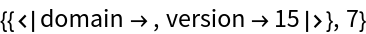Import the model back into Wolfram Language. However, the NetEncoder and NetDecoder will be absent because they are not supported by ONNX:

 In:=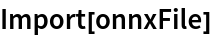Out=